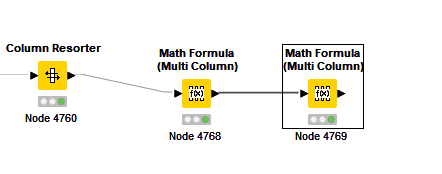# Math Formula Multi Column, Column Average

I simply want to calculate the average value in each column of the Math Formula Multi Column node–but not allow zeros in the cells to reduce the average value of the column.

For example, see column 4 below. I don’t want all the zeros to take away from the value of 398.14. So if 398.14 and 402.68 are the only two non-zero values in the column I want my calculated average value to be 398.14 + 402.68 = 400.68, not some tiny number once all of the zeros in the column are added in.

While the number of rows is the same, the number of non-zero values vary from 1 to 1600. So I can’t just set a standard and divide each column’s sum.

I feel stupid, but after half a day I just cannot figure out how to make the Math Formula do this.

Thanks

Hi @creedsmith check this post: Calculate mean ignoring zeros. First convert the zeros to NaN with ```
if(\$CURRENT_COLUMN\$ == 0, “NaN”, \$CURRENT_COLUMN\$)

``and then use COLMEAN``
2 Likes

Hi @creedsmith -

There’s an approach you can use with the Rule Engine here. If you set up an expression like

`\$mycolumn\$ > 0 => \$mycolumn\$`

Then all values greater than zero will retain their value, and all values of zero will be converted to missing (the red question mark in KNIME). Then when you calculate the average of that column in the Math Formula, the missings are ignored.

A bit hacky, but works fineEDIT: And now I see @iperez beat me to it with a better solution. Nice!

2 Likes

thank you so very much

thank you very much. always nice to see how my stupidity can be cured in ten seconds

1 Like

I blew something up. Please see my setting and results (results should be closer to say 489.65 or something–not in the tens of thousands)

is this more like what you were thinking: tow different processes using math formula multi columnand then seeing results something like this

Hi @creedsmith . I was thinking on the second approach. Does that work?

yep, doing the steps as two different calculations works out. thanks again

2 Likes

have you tried wrapping the col_mean around the if condition? Just wondering if you could do it in one expression but not sure
bR

Hi Daniel, I did try, but must have had an error in my logic. the outcome blew up on me. thanks for the input.

This topic was automatically closed 182 days after the last reply. New replies are no longer allowed.